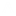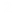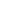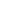```   1 {-
2
3 Pearl 4: Selection problem
4
5 Definitions:
6 X and Y two finite disjoint sets, sorted.
7
8 Problem:
9 Find the k-smallest elemnt in the set (X union Y)
10
11 Solution:
12 You can do it of course in linear time O(K).
13 But it turns out you can do faster by divide and conquer.
14 The trick is to do the merge-decisions in the right way.
15
16 We then also use arrays rather than lists, to get efficency in time.
17
18 <== And this is where it seems the book messed up on indices. Corrected in my code.
19
20 I left all the debugging and printing in there, so one can see the equivalence between
21 Array-indices and Lists.
22
23 -}
24 module P4 where
25
26 import Data.Array   (Array, listArray, bounds, (!))
27 import Debug.Trace
28
29 -- Test function(s)
30 {-
31 x = [7,14..28]
32 y = [5,10..35]
33 k = 4
34 -}
35 y = [7,14..28]
36 x = [5,10..35]
37 --k = 4
38 k = 7
39
40 xa = listArray (0,length x-1) x
41 ya = listArray (0,length y-1) y
42
43 -- Brute force
44 -- Requires (z+1)^2 evaluations of f
45 smallest1             ::     Ord a => Int -> ([a],[a]) -> a
46 smallest1 k (xs,ys)  =     union (xs,ys) !!k
47
48 union (xs,[])        =     xs
49 union ([],ys)        =     ys
50 union (x:xs,y:ys)    |    x < y     = x : union(xs,y:ys)
51                     |     x > y     = y : union(x:xs,ys)
52
53
54
55 -- Divide and Conquer
56 smallest2 k ([],ws)    = ws !! k
57 smallest2 k (zs,[])    = zs !! k
58 smallest2 k (zs,ws)    =
59     trace  ("zs=" ++ show zs ++
60     "  and  ws=" ++ show ws  ++
61     " and k=" ++ show k ++ "\n" ++
62     " and (p,q)=" ++ show (p,q) ++
63     "and (a,b)=" ++ show(a,b) )\$
64     case (a < b, k <= p+q) of
65         (True,True)   -> trace "--> (1)" \$ smallest2 k (zs,us)
66         (True,False)  -> trace "--> (2)" \$ smallest2 (k-p-1) (ys,ws)
67         (False,True)  -> trace "--> (3)" \$ smallest2 k (xs,ws)
68         (False,False) -> trace "--> (4)" \$ smallest2 (k-q-1) (zs,vs)
69     where
70         p               = (length zs) `div` 2
71         q               = (length ws) `div` 2
72         (xs,a:ys)      = splitAt p zs
73         (us,b:vs)      = splitAt q ws
74
75
76 -- Divide and Conquer, using arrays
77
78 smallest3             ::     Ord a => Show a => Int -> (Array Int a, Array Int a) -> a
79 smallest3 k (xa,ya)    =     search k (0,m+1) (0,n+1)
80                         where
81                             (0,m) = bounds xa
82                             (0,n) = bounds ya
83                             -- The below is actually writing smallest2, but using array notation!
84                             search k (lx,rx) (ly,ry)
85                                 | lx==rx     = ya ! ly     -- x = []
86                                 | ly==ry     = xa ! lx     -- y = []
87                                 | otherwise    =     trace  ("(lx,rx)=" ++ show (lx,rx) ++
88                                                 "  and  (ly,ry)=" ++ show (ly,ry)  ++
89                                                 " and k=" ++ show k ++ "\n" ++
90                                                 " and (mx,my)=" ++ show (mx,my) ++
91                                                 " and (mx',my')=" ++ show (mx',my') ++
92                                                 "and (xa!mx,ya!my)=" ++ show(xa!mx,ya!my) )\$
93                                                 case ( (xa!mx) < (ya!my), k <= mx'+my') of
94                                                 (True,True)   -> trace "--> (1)" \$ search k (lx,rx) (ly,my)
95                                                 (True,False)  -> trace "--> (2)" \$ search (k-mx'-1) (mx+1,rx) (ly,ry)
96                                                 (False,True)  -> trace "--> (3)" \$ search k (lx,mx) (ly,ry)
97                                                 (False,False) -> trace "--> (4)" \$ search (k-my'-1) (lx,rx) (my+1,ry)
98                                             where
99                                                 mx               = (lx + rx) `div` 2
100                                                 my               = (ly + ry) `div` 2
101                                                 mx'           = (rx - lx) `div` 2
102                                                 my'           = (ry - ly) `div` 2
103
104 -- Main
105
106 main = do
107     print \$ "x=" ++ (show x)
108     print \$ "y=" ++ (show y)
109     print \$ "union (x,y)=" ++ (show \$ union (x,y))
110     print \$ "Looking for element : " ++ (show k) ++ " (remember, indexing starts from 0)"
111     putStr "Brute force                   : "
112     print \$ smallest1 k (x,y)
113     putStrLn "Divide-and-Conquer (w/ lists) : "
114     print \$ smallest2 k (x,y)
115     putStr "Divide-and-Conquer (w/ arrays): "
116     print \$ smallest3 k (xa,ya)
```